# 共享智能指针

## 1、std::shared_ptr的初始化

``````// 管理当前对象的 shared_ptr 实例数量，或若无被管理对象则为 0。
long use_count() const noexcept;
``````

### 1.1、通过构造函数初始化

``````// shared_ptr<T> 类模板中，提供了多种实用的构造函数, 语法格式如下:
std::shared_ptr<T> 智能指针名字(创建堆内存);
``````

``````#include <iostream>
#include <memory>
using namespace std;

int main()
{
// 使用智能指针管理一块 int 型的堆内存
shared_ptr<int> ptr1(new int(520));
cout << "ptr1.use_count: " << ptr1.use_count() << endl;
// 使用智能指针管理一块字符数组对应的堆内存
shared_ptr<char> ptr2(new char);
cout << "ptr2.use_count: " << ptr2.use_count() << endl;
// 创建智能指针对象, 不管理任何内存
shared_ptr<int> ptr3;
cout << "ptr3.use_count: " << ptr3.use_count() << endl;
// 创建智能指针对象, 初始化为空
shared_ptr<int> ptr4(nullptr);
cout << "ptr4.use_count: " << ptr4.use_count() << endl;

return 0;
}
``````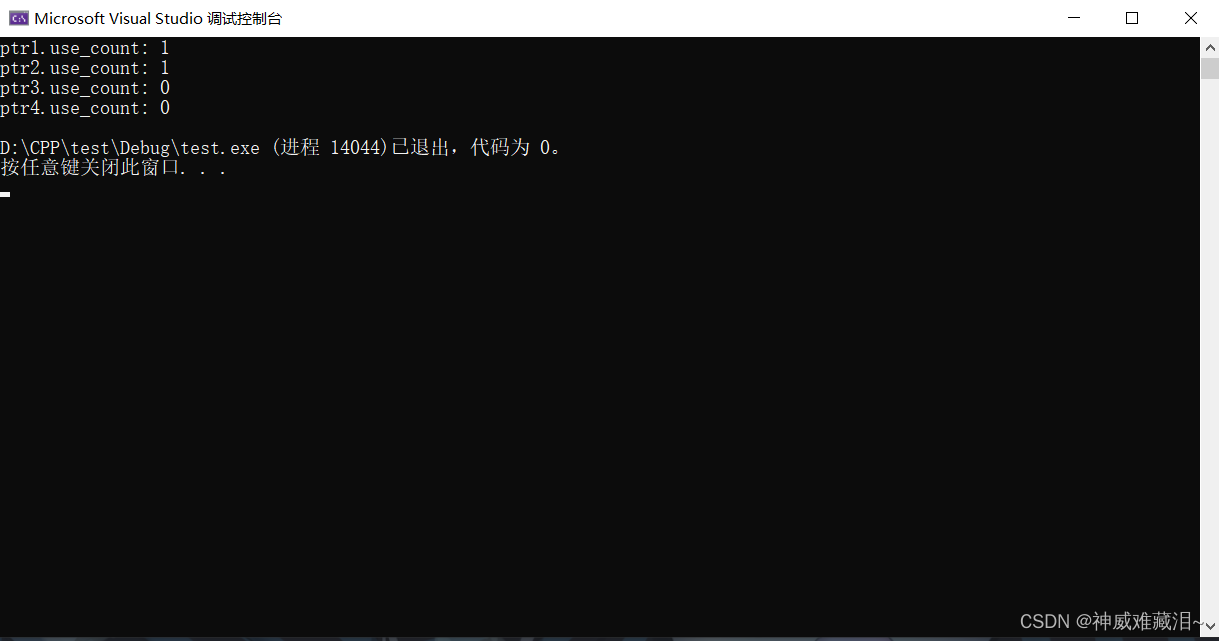``````int *p = new int;
shared_ptr<int> p1(p);
shared_ptr<int> p2(p);		// error, 编译不会报错, 运行会出错，指针p已经初始化了p1了
``````

### 1.2、通过拷贝和移动构造函数初始化

``````#include <iostream>
#include <memory>
using namespace std;

int main()
{
// 使用智能指针管理一块 int 型的堆内存, 内部引用计数为 1
shared_ptr<int> ptr1(new int(520));
cout << "ptr1管理的内存引用计数: " << ptr1.use_count() << endl;
//调用拷贝构造函数
shared_ptr<int> ptr2(ptr1);
cout << "ptr2管理的内存引用计数: " << ptr2.use_count() << endl;
shared_ptr<int> ptr3 = ptr1;
cout << "ptr3管理的内存引用计数: " << ptr3.use_count() << endl;
//调用移动构造函数
shared_ptr<int> ptr4(std::move(ptr1));
cout << "ptr4管理的内存引用计数: " << ptr4.use_count() << endl;
std::shared_ptr<int> ptr5 = std::move(ptr2);
cout << "ptr5管理的内存引用计数: " << ptr5.use_count() << endl;

return 0;
}
``````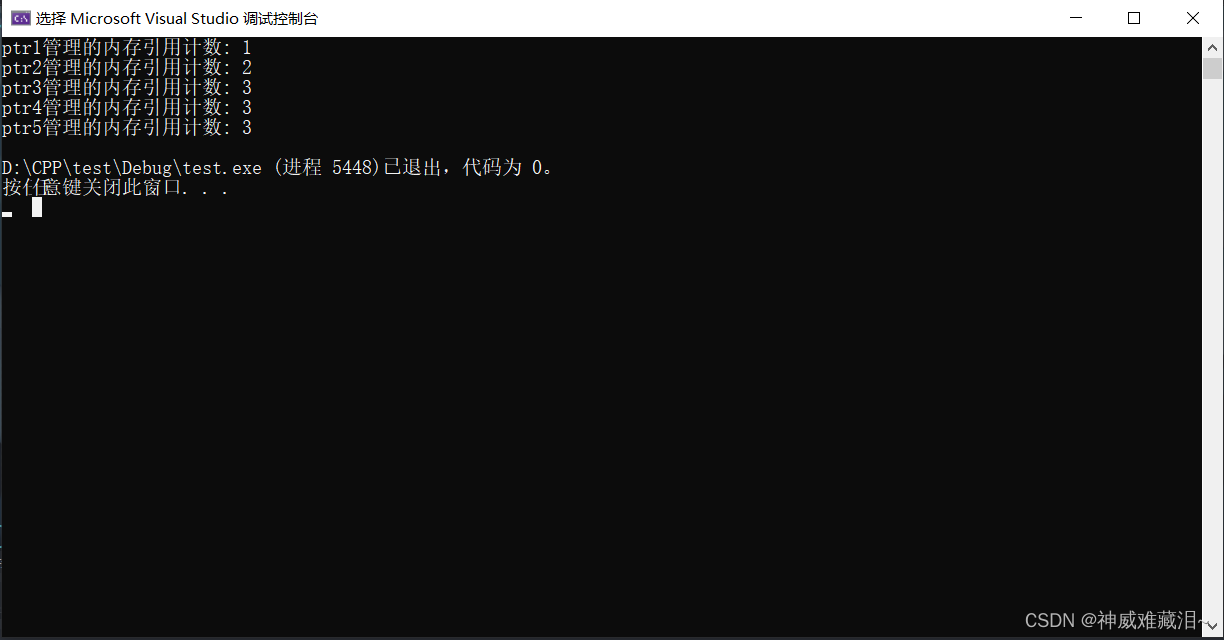### 1.3、通过std::make_shared初始化

``````template< class T, class... Args >
shared_ptr<T> make_shared( Args&&... args );
``````
• T:模板参数类型。
• Arg&&…arg：要初始化的数据，如果是通过make_shared创建对象，需要按照构造函数的参数列表指定。

``````#include <iostream>
#include <string>
#include <memory>
using namespace std;

class Test
{
public:
Test()
{
cout << "construct Test..." << endl;
}
Test(int x)
{
cout << "construct Test, x = " << x << endl;
}
Test(string str)
{
cout << "construct Test, str = " << str << endl;
}
~Test()
{
cout << "destruct Test ..." << endl;
}
};

int main()
{
// 使用智能指针管理一块 int 型的堆内存, 内部引用计数为 1
shared_ptr<int> ptr1 = make_shared<int>(520);
cout << "ptr1管理的内存引用计数: " << ptr1.use_count() << endl;
//使用智能指针管理一块无参Test类类型的内存，内部引用计数为1
shared_ptr<Test> ptr2 = make_shared<Test>();
cout << "ptr2管理的内存引用计数: " << ptr2.use_count() << endl;
//使用智能指针管理一块带有int类型参数的Test类类型的内存，内部引用计数为1
shared_ptr<Test> ptr3 = make_shared<Test>(520);
cout << "ptr3管理的内存引用计数: " << ptr3.use_count() << endl;
//使用智能指针管理一块string类型参数的Test类类型的内存，内部引用计数为1
shared_ptr<Test> ptr4 = make_shared<Test>("Naruto");
cout << "ptr4管理的内存引用计数: " << ptr4.use_count() << endl;
return 0;
}
``````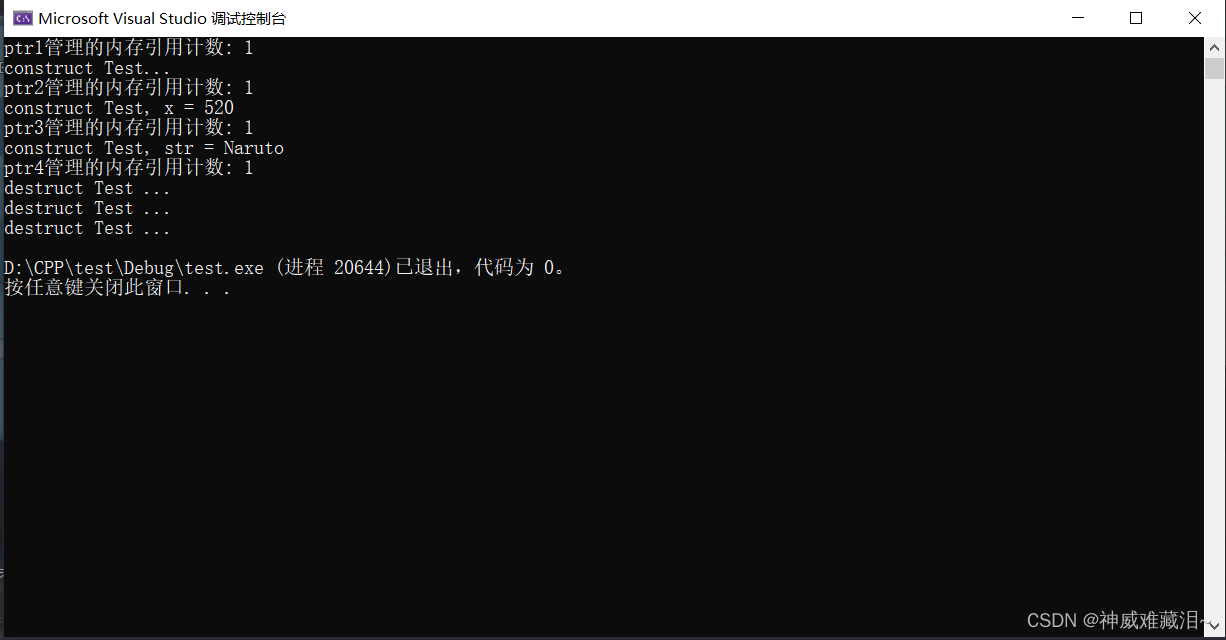### 1.4、通过reset方法初始化

``````void reset() noexcept;

template< class Y >
void reset( Y* ptr );

template< class Y, class Deleter >
void reset( Y* ptr, Deleter d );

template< class Y, class Deleter, class Alloc >
void reset( Y* ptr, Deleter d, Alloc alloc );
``````
• ptr：指向要取得所有权的对象的指针。
• d：指向要取得所有权的对象的删除器。
• aloc：内部存储所用的分配器。

``````#include <iostream>
#include <string>
#include <memory>
using namespace std;

int main()
{
// 使用智能指针管理一块 int 型的堆内存, 内部引用计数为 1
shared_ptr<int> ptr1 = make_shared<int>(520);
//拷贝赋值，引用计数加1
shared_ptr<int> ptr2 = ptr1;
shared_ptr<int> ptr3 = ptr1;
shared_ptr<int> ptr4 = ptr1;
cout << "ptr1.use_count: " << ptr1.use_count() << endl;
cout << "ptr2.use_count: " << ptr2.use_count() << endl;
cout << "ptr3.use_count: " << ptr3.use_count() << endl;
cout << "ptr4.use_count: " << ptr4.use_count() << endl;
cout << endl;
//释放智能指针指向的内存，清空引用计数
ptr4.reset();
cout << "ptr1.use_count: " << ptr1.use_count() << endl;
cout << "ptr2.use_count: " << ptr2.use_count() << endl;
cout << "ptr3.use_count: " << ptr3.use_count() << endl;
cout << "ptr4.use_count: " << ptr4.use_count() << endl;
cout << endl;
shared_ptr<int> ptr5;
//初始化智能指针，引用计数加1
ptr5.reset(new int(250));
cout << "ptr5.use_count: " << ptr5.use_count() << endl;

return 0;
}
``````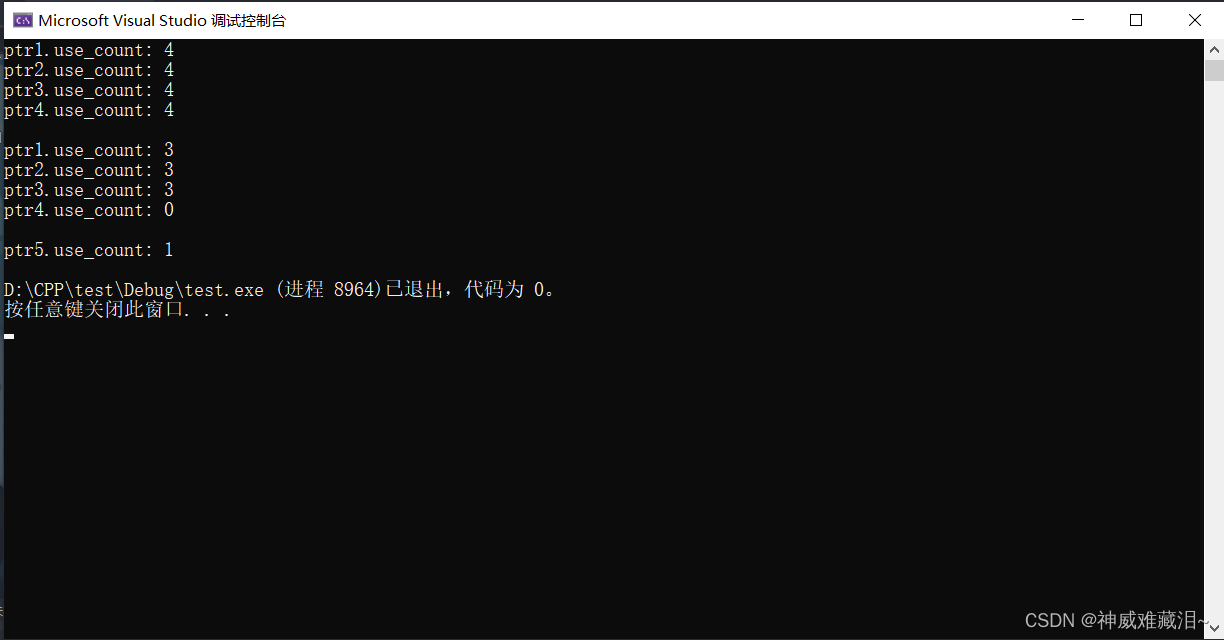## 2、获取原始指针

``````T* get() const noexcept;
``````

``````#include <iostream>
#include <string>
#include <memory>
using namespace std;

int main()
{
int len = 128;
shared_ptr<char> ptr(new char[len]);
// 得到指针的原始地址
//初始化字符串
//字符串拷贝
strcpy(add, "I will be Naruto !!!");
cout << "string: " << add << endl;

shared_ptr<int> p(new int);
*p = 100;
cout << *p.get() << "  " << *p << endl;

return 0;
}
``````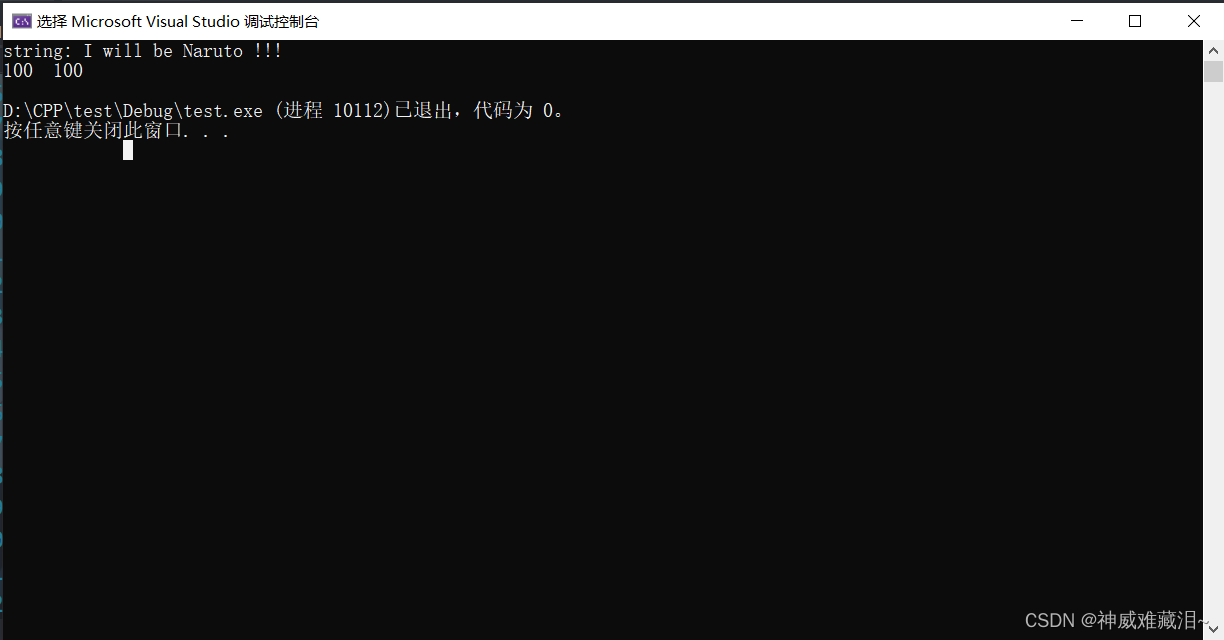THE END